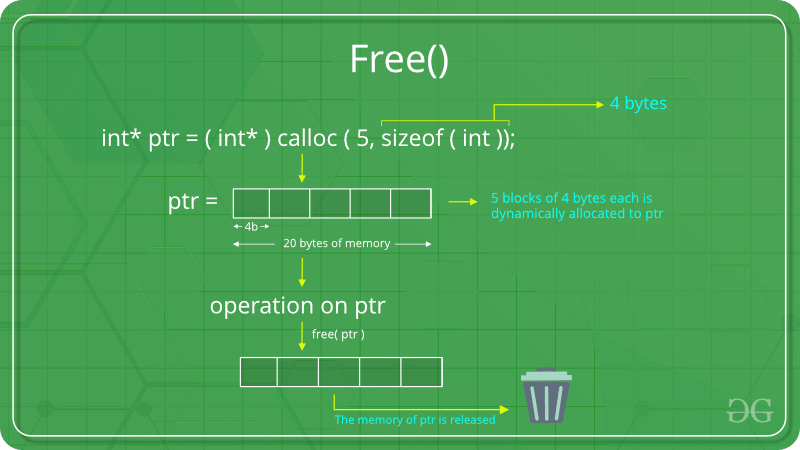# free() Function in C Library With Examples

• Difficulty Level : Medium
• Last Updated : 05 Aug, 2022

When memory blocks are allotted by calloc(), malloc(), or realloc()  functions, the C library function free() is used to deallocate or release the memory blocks to reduce their wastage.

free() function in C should only be used either for the pointers pointing to the memory allocated using malloc() or for a NULL pointer. free() function only frees the memory from the heap and it does not call the destructor. To destroy the allocated memory and call the destructor we can use the delete() operator in C.Syntax to Use free() function in C

`void free(void *ptr)`

Here, ptr is the memory block that needs to be freed or deallocated.

For example, program 1 demonstrates how to use free() with calloc() in C and program 2 demonstrates how to use free() with malloc() in C.

Program 1:

## C

 `// C program to demonstrate use of ``// free() function using calloc()``#include ``#include ``int` `main()``{`` ` `    ``// This pointer ptr will hold the``    ``// base address of the block created``    ``int``* ptr;``    ``int` `n = 5;`` ` `    ``// Get the number of elements for the array``    ``printf``(``"Enter number of Elements: %d\n"``, n);`` ` `    ``scanf``(``"%d"``, &n);`` ` `    ``// Dynamically allocate memory using calloc()``    ``ptr = (``int``*)``calloc``(n, ``sizeof``(``int``));`` ` `    ``// Check if the memory has been successfully``    ``// allocated by calloc() or not``    ``if` `(ptr == NULL) {``        ``printf``(``"Memory not allocated \n"``);``        ``exit``(0);``    ``}``    ``// Memory has been Successfully allocated using calloc()``    ``printf``(``"Successfully allocated the memory using calloc(). \n"``);`` ` `    ``// Free the memory``    ``free``(ptr);`` ` `    ``printf``(``"Calloc Memory Successfully freed."``);`` ` `    ``return` `0;``}`

Output

```Enter number of Elements: 5
Successfully allocated the memory using calloc().
Calloc Memory Successfully freed.```

Program 2:

## C

 `// C program to demonstrate use of``// free() function using malloc()``#include ``#include `` ` `int` `main()``{`` ` `    ``// This pointer ptr will hold the``    ``// base address of the block created``    ``int``* ptr;``    ``int` `n = 5;`` ` `    ``// Get the number of elements for the array``    ``printf``(``"Enter number of Elements: %d\n"``, n);`` ` `    ``scanf``(``"%d"``, &n);`` ` `    ``// Dynamically allocate memory using malloc()``    ``ptr = (``int``*)``malloc``(n * ``sizeof``(``int``));`` ` `    ``// Check if the memory has been successfully``    ``// allocated by malloc() or not``    ``if` `(ptr == NULL) {``        ``printf``(``"Memory not allocated \n"``);``        ``exit``(0);``    ``}``    ``// Memory has been Successfully allocated using malloc()``    ``printf``(``"Successfully allocated the memory using malloc(). \n"``);`` ` `    ``// Free the memory``    ``free``(ptr);`` ` `    ``printf``(``"Malloc Memory Successfully freed."``);`` ` `    ``return` `0;``}`

Output

```Enter number of Elements: 5
Successfully allocated the memory using malloc().
Malloc Memory Successfully freed.```

My Personal Notes arrow_drop_up